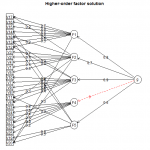Want to share your content on R-bloggers? click here if you have a blog, or here if you don't.This set of exercises is about exploratory factor analysis. We shall use some basic features of `psych` package. For quick introduction to exploratory factor analysis and `psych` package, we recommend this short “how to” guide.

Answers to the exercises are available here.

If you have different solution, feel free to post it.

Exercise 1

Load the data, install the packages `psych` and `GPArotation` which we will use in the following exercises, and load it. Describe the data.

Exercise 2

Using the parallel analysis, determine the number of factors.

Exercise 3

Determine the number of factors using Very Simple Structure method.

Exercise 4

Based on normality test, is the Maximum Likelihood factoring method proper, or is OLS/Minres better? (Tip: Maximum Likelihood method requires normal distribution.)

Exercise 5

Using oblimin rotation, 5 factors and factoring method from the previous exercise, find the factor solution. Print loadings table with cut off at 0.3.

Exercise 6

Exercise 7

Plot structure diagram.

Exercise 8

Find the higher-order factor model with five factors plus general factor.

Exercise 9

Find the bifactor solution.

Exercise 10

Reduce the number of dimensions using hierarchical clustering analysis.